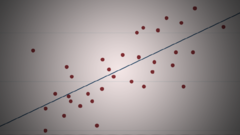## Applied Regression Analysis

 所在平台: Coursera 课程类别: 统计和数据分析 授课老师： Stanley LemeshowExplore 1600+ online courses from top universities. Join Coursera today to learn data science, programming, business strategy, and more.#### 课程详情

Statistical modeling is a fundamental element of analysis for statisticians, epidemiologists, biostatisticians and other professionals of related disciplines. People in the health sciences profession rely on regression modeling to gain insight on make decisions based on a continuous flow of response data.

Focusing on linear and multiple regression, this course will provide theoretical and practical training in statistical modeling.

This is a hands-on, applied course where students will become proficient at using computer software to analyze data drawn primarily from the fields of medicine, epidemiology and public health.

There will be many practical examples and homework exercises in this class to help you learn. If you fully apply yourself in this course and complete all of the homework, you will have the opportunity to master methods of statistical modeling when the response variable is continuous and you will become a confident user of the Stata* package for computing linear, polynomial and multiple regression.

*Access to Stata will be provided at no cost for the duration of this course.

#### 课程大纲

Week One
• Review of basic statistical concepts
• Regression and correlation
Week Two
• Linear regression
• Assumptions for linear regression
• Hypothesis test and confidence intervals for model parameters
Week Three
• The correlation coefficient
• The ANOVA table for straight line regression
Week Four
• Polynomial regression
Week Five
• Multiple regression
• The partial F-test
Week Six
• Dummy (or indicator) variables
• Statistical interaction
• Comparing two straight line regression equations

#### 欢迎关注我们的公众号#### 课程简介

Regression modeling is the standard method for analysis of continuous response data. This course provides theoretical and practical training in statistical modeling with particular emphasis on linear and multiple regression.

ANOVA 统计学 回归分析

#### 4人关注该课程